The website uses cookies to optimize your user experience. Using this website grants us the permission to collect certain information essential to the provision of our services to you, but you may change the cookie settings within your browser any time you wish. Learn more
blank_error__body
Text direction?

# Log-likelihood

by Marco Taboga, PhD

The log-likelihood is, as the term suggests, the natural logarithm of the likelihood.

In turn, given a sample and a parametric family of distributions (i.e., a set of distributions indexed by a parameter) that could have generated the sample, the likelihood is a function that associates to each parameter the probability (or probability density) of observing the given sample.## Definition

The following elements are needed to rigorously define the log-likelihood function:

• we observe a sample, which is regarded as the realization of a random vector, whose distribution is unknown;

• the distribution ofbelongs to a parametric family: there is a setof real vectors (called the parameter space) whose elements (called parameters) are put into correspondence with the distributions that could have generated; in particular:

• ifis an continuous random vector, its joint probability density function belongs to a set of joint probability density functionsindexed by the parameter;

• ifis a discrete random vector, its joint probability mass function belongs to a set of joint probability mass functionsindexed by the parameter;

• when the joint probability mass (or density) function is considered as a function offor fixed(i.e., for the samplewe have observed), it is called likelihood (or likelihood function) and it is denoted by. So,ifis discrete andifis continuous.

Given all these elements, the log-likelihood function is the functiondefined by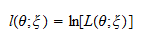## Example

The typical example is the log-likelihood function of a sample that is made up of independent and identically distributed draws from a normal distribution.

In this case, the sampleis a vector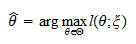whose entriesare draws from a normal distribution. The probability density function of a generic drawis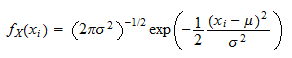whereandare the parameters (mean and variance) of the normal distribution.

With the notation used in the previous section, the parameter vector isThe parametric family being considered is the set of all normal distributions (that can be obtained by varying the parametersand).

In order to stress the fact that the probability density depends on the two parameters, we write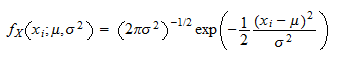The joint probability density of the sampleis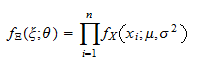because the joint density of a set of independent variables is equal to the product of their marginal densities (see the lecture on Independent random variables).

The likelihood function is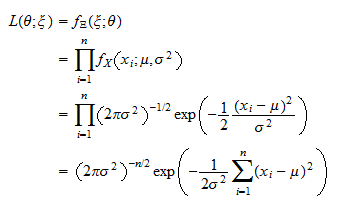The log-likelihood function is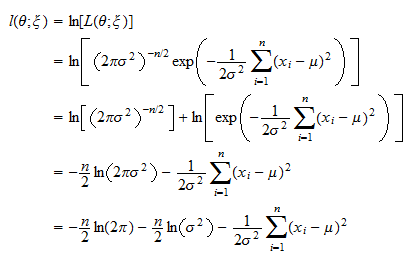## How the log-likelihood is used

The log-likelihood function is typically used to derive the maximum likelihood estimator of the parameter. The estimatoris obtained by solvingthat is, by finding the parameterthat maximizes the log-likelihood of the observed sample. This is the same as maximizing the likelihood functionbecause the natural logarithm is a strictly increasing function.

## Why the log is taken

One may wonder why the log of the likelihood function is taken. There are several good reasons. To understand them, suppose that the sample is made up of independent observations (as in the example above). Then, the logarithm transforms a product of densities into a sum. This is very convenient because:

• the asymptotic properties of sums are easier to analyze (one can apply Laws of Large Numbers and Central Limit Theorems to these sums; see the proofs of consistency and asymptotic normality of the maximum likelihood estimator);

• products are not numerically stable: they tend to converge quickly to zero or to infinity, depending on whether the densities of the single observations are on average less than or greater than 1; sums are instead more stable from a numerical standpoint; this is important because the maximum likelihood problem is often solved numerically on computers where limited machine precision does not allow to distinguish a very small number from zero and a very large number from infinity.

## More examples

More example of how to derive log-likelihood functions can be found in the lectures on:

## More details

The log-likelihood and its properties are discussed in a more detailed manner in the lecture on maximum likelihood estimation.

Previous entry: Joint probability mass function

Next entry: Loss function

## How to cite

Taboga, Marco (2017). "Log-likelihood", Lectures on probability theory and mathematical statistics, Third edition. Kindle Direct Publishing. Online appendix. https://www.statlect.com/glossary/log-likelihood.

Measure
Measure
Related Notes
Get a free MyMarkup account to save this article and view it later on any device.
Create account

Summary | 8 Annotations
the likelihood is a function that associates to each parameter the probability (or probability density) of observing the given sample
2020/08/12 08:22
a sample
2020/08/12 08:22
e ,
2020/08/12 08:22
realization of a random vector ,
2020/08/12 08:22
distribution of belongs to a parametric family: there is a set of real vectors (called the parameter space) whose elements (called parameters) are put into correspondence with the distributions that could have generated ;
2020/08/12 08:23
a function of for fixed (i.e., for the sample we have observed), it is called likelihood (or likelihood function) and it is denoted by .
2020/08/12 08:24
log-likelihood function is the function defined by
2020/08/12 08:24
by finding the parameter that maximizes the log-likelihood of the observed sample . This is the same as maximizing the likelihood function
2020/08/12 08:27